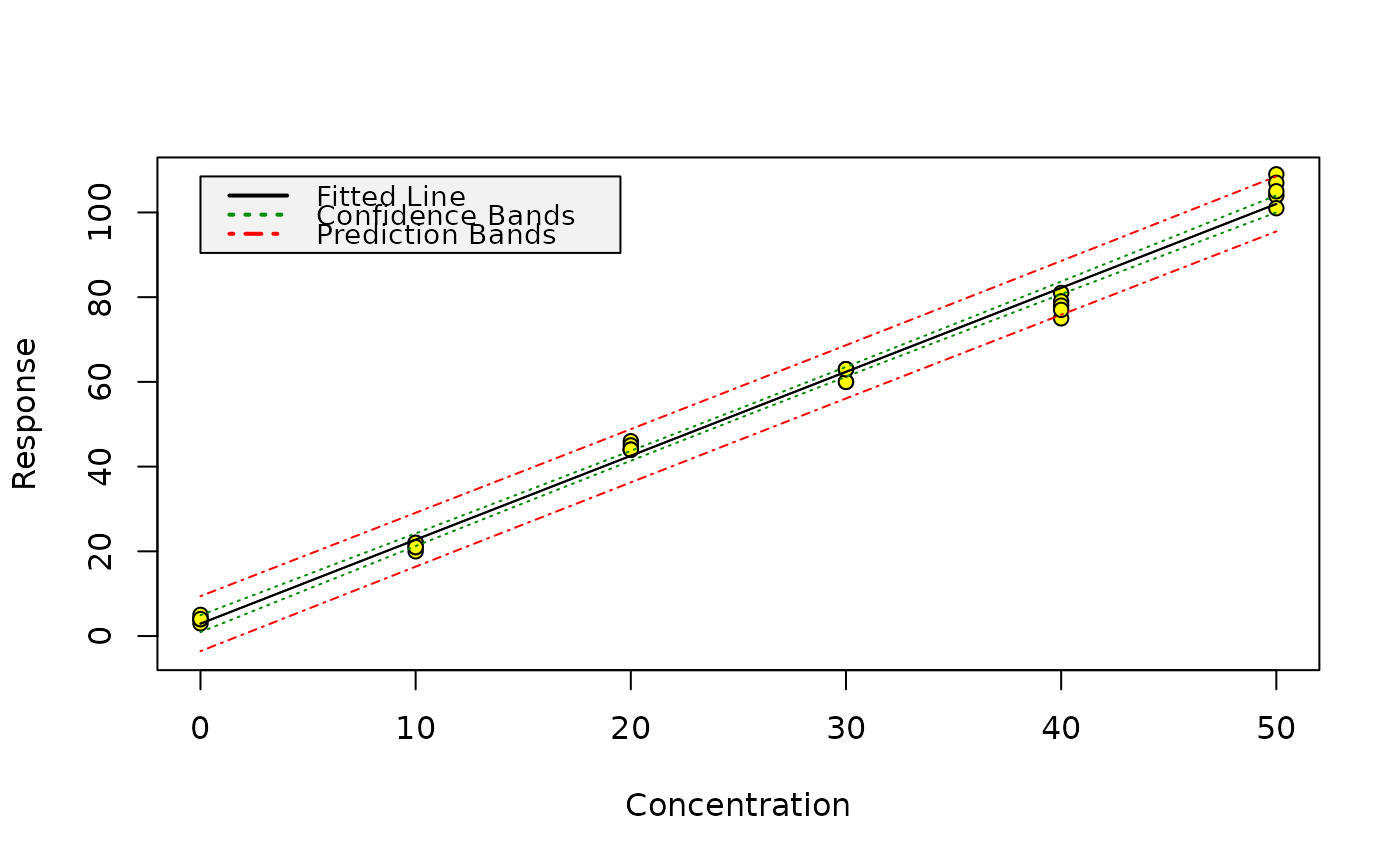Produce graphics of calibration data, the fitted model as well as confidence, and, for unweighted regression, prediction bands.

calplot(object, xlim = c("auto", "auto"), ylim = c("auto", "auto"),
xlab = "Concentration", ylab = "Response", legend_x = "auto",
alpha=0.05, varfunc = NULL)

## Arguments

object A univariate model object of class lm or rlm with model formula y ~ x or y ~ x - 1. The limits of the plot on the x axis. The limits of the plot on the y axis. The label of the x axis. The label of the y axis. An optional numeric value for adjusting the x coordinate of the legend. The error tolerance level for the confidence and prediction bands. Note that this includes both tails of the Gaussian distribution, unlike the alpha and beta parameters used in lod (see note below). The variance function for generating the weights in the model. Currently, this argument is ignored (see note below).

## Value

A plot of the calibration data, of your fitted model as well as lines showing the confidence limits. Prediction limits are only shown for models from unweighted regression.

## Note

Prediction bands for models from weighted linear regression require weights for the data, for which responses should be predicted. Prediction intervals using weights e.g. from a variance function are currently not supported by the internally used function predict.lm, therefore, calplot does not draw prediction bands for such models.

It is possible to compare the calplot prediction bands with the lod values if the lod() alpha and beta parameters are half the value of the calplot() alpha parameter.

## Author

Johannes Ranke jranke@uni-bremen.de

## Examples

data(massart97ex3)
m <- lm(y ~ x, data = massart97ex3)
calplot(m)